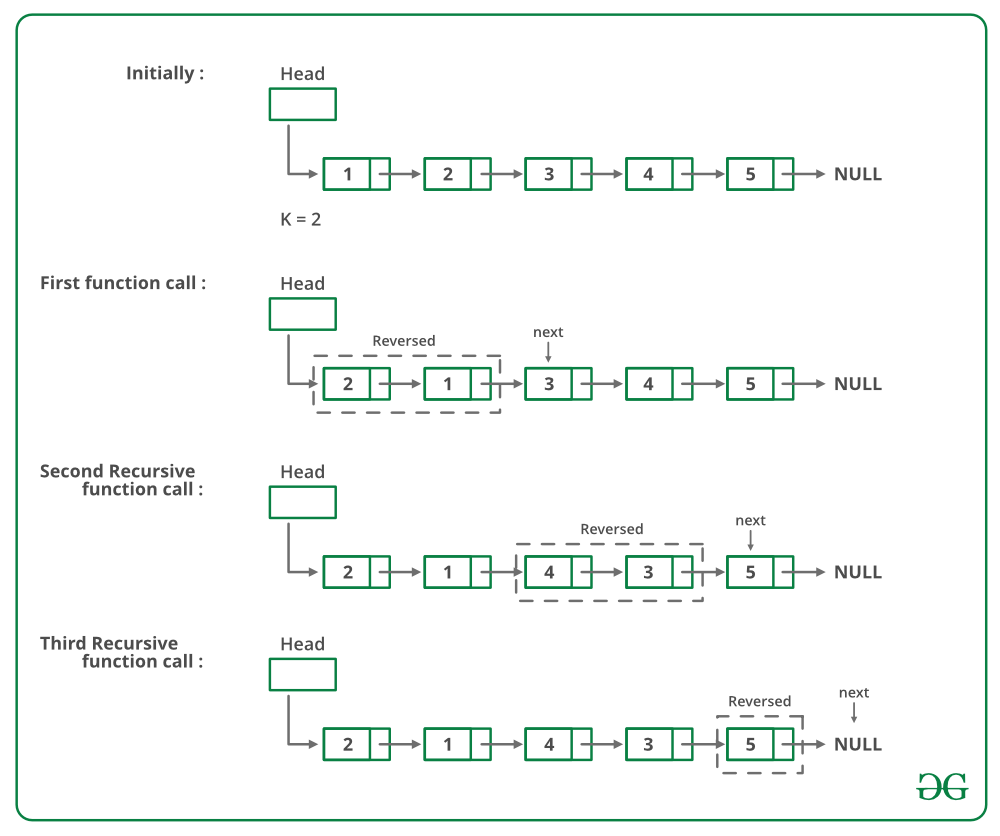GeeksforGeeks App
Open AppBrowser
Continue

# Python Program For Reversing A Linked List In Groups Of Given Size – Set 1

Given a linked list, write a function to reverse every k nodes (where k is an input to the function).

Example:

Input: 1->2->3->4->5->6->7->8->NULL, K = 3
Output: 3->2->1->6->5->4->8->7->NULL
Input: 1->2->3->4->5->6->7->8->NULL, K = 5
Output: 5->4->3->2->1->8->7->6->NULL

• Reverse the first sub-list of size k. While reversing keep track of the next node and previous node. Let the pointer to the next node be next and pointer to the previous node be prev. See this post for reversing a linked list.
• head->next = reverse(next, k) ( Recursively call for rest of the list and link the two sub-lists )
• Return prev ( prev becomes the new head of the list (see the diagrams of an iterative method of this post )

Below is image shows how the reverse function works:Below is the implementation of the above approach:

## Python

 `# Python program to reverse a ``# linked list in group of given size`` ` `# Node class``class` `Node:`` ` `    ``# Constructor to initialize the ``    ``# node object``    ``def` `__init__(``self``, data):``        ``self``.data ``=` `data``        ``self``.``next` `=` `None`` ` ` ` `class` `LinkedList:`` ` `    ``# Function to initialize head``    ``def` `__init__(``self``):``        ``self``.head ``=` `None`` ` `    ``def` `reverse(``self``, head, k):      ``        ``if` `head ``=``=` `None``:``          ``return` `None``        ``current ``=` `head``        ``next` `=` `None``        ``prev ``=` `None``        ``count ``=` `0`` ` `        ``# Reverse first k nodes of the linked list``        ``while``(current ``is` `not` `None` `and` `              ``count < k):``            ``next` `=` `current.``next``            ``current.``next` `=` `prev``            ``prev ``=` `current``            ``current ``=` `next``            ``count ``+``=` `1`` ` `        ``# next is now a pointer to (k+1)th node``        ``# recursively call for the list starting``        ``# from current. And make rest of the list as``        ``# next of first node``        ``if` `next` `is` `not` `None``:``            ``head.``next` `=` `self``.reverse(``next``, k)`` ` `        ``# prev is new head of the input list``        ``return` `prev`` ` `    ``# Function to insert a new node at ``    ``# the beginning``    ``def` `push(``self``, new_data):``        ``new_node ``=` `Node(new_data)``        ``new_node.``next` `=` `self``.head``        ``self``.head ``=` `new_node`` ` `    ``# Utility function to print the ``    ``# Linked List``    ``def` `printList(``self``):``        ``temp ``=` `self``.head``        ``while``(temp):``            ``print` `temp.data,``            ``temp ``=` `temp.``next`` ` `# Driver code``llist ``=` `LinkedList()``llist.push(``9``)``llist.push(``8``)``llist.push(``7``)``llist.push(``6``)``llist.push(``5``)``llist.push(``4``)``llist.push(``3``)``llist.push(``2``)``llist.push(``1``)`` ` `print` `"Given linked list"``llist.printList()``llist.head ``=` `llist.reverse(llist.head, ``3``)`` ` `print` `"Reversed Linked list"``llist.printList()``# This code is contributed by Nikhil Kumar Singh(nickzuck_007)`

Output:

```Given Linked List
1 2 3 4 5 6 7 8 9
Reversed list
3 2 1 6 5 4 9 8 7 ```

Complexity Analysis:

• Time Complexity: O(n).
Traversal of list is done only once and it has ‘n’ elements.
• Auxiliary Space: O(n/k).
For each Linked List of size n, n/k or (n/k)+1 calls will be made during the recursion.

Please refer complete article on Reverse a Linked List in groups of given size | Set 1 for more details!

My Personal Notes arrow_drop_up